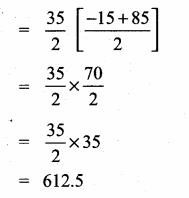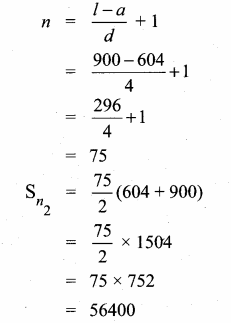# Samacheer Kalvi 10th Maths Guide Chapter 2 Numbers and Sequences Ex 2.6.

23 Nov 2020 9:02 am

Tamilnadu, Samacheer Kalvi, 10th, Maths, Solutions, Chapter 2, Numbers and Sequences, Ex 2.6,

Question 1.
Find the sum of the following
(i) 3, 7, 11,… up to 40 terms.
3,7,11,… up to 40 terms
First term (a) = 3
Common difference (d) = 7 – 3 = 4
Number of terms (n) = 40
Sn = n2 [2a + (n – 1) d]
S40 = 402 [6 + 39 × 4] = 20 [6 + 156]
= 20 × 162
S40 = 3240

(ii) 102,97, 92,… up to 27 terms.
Here a = 102, d = 97 – 102 = -5
n = 27
Sn = n2 [2a + (n – 1)d]
S27 = 272 [2(102) + 26(-5)]
= 272 [204 – 130]
= 272 × 74
= 27 × 37 = 999
S27 = 999

(iii) 6 + 13 + 20 + …. + 97
Here a = 6, d = 13 – 6 = 7, l = 97
= 97−67 + 1
= 917 + 1 =
13 + 1 = 14
Sn = n2 (a + l)
Sn = 142 (a + l)
Sn = 142 (6 + 97)
= 7 × 103
Sn = 721

Question 2.
How many consecutive odd integers beginning with 5 will sum to 480?
First term (a) = 5
Common difference (d) = 2
(consecutive odd integer)
Sn = 480
n2 [2a + (n-1) d] = 480
n2 [10 + (n-1)2] = 480

n + 24 = 0 or n – 20 = 0
n = -24 or n = 20
[number of terms cannot be negative]
∴ Number of consecutive odd integers is 20

Question 3.
Find the sum of first 28 terms of an A.P. whose nth term is 4n – 3.
Solution:
n = 28
tn = 4n – 3
t1 = 4 × 1 – 3 = 1
t2 = 4 × 2 – 3 = 5
t28 = 4 × 28 – 3
= 112 – 3 = 109
∴ a = 1, d = t2 – t1 = 5 – 1 = 4
l = 109.
Sn = n2 (2a+(n – 1)d)
S28 = 282 (2 × 1 + 27 × 4)
= 14(2 + 108)
= 14 × 110
= 1540

Question 4.
The sum of first n terms of a certain series is given as 2n2 – 3n . Show that the series is an A.P.
Let tn be nth term of an A.P.
tn = Sn – Sn-1
= 2n2 – 3n – [2(n – 1)2 – 3(n – 1)]
= 2n2 – 3n – [2(n2 – 2n + 1) – 3n + 3]
= 2n2 – 3n – [2n2 – 4n + 2 – 3n + 3]
= 2n2 – 3n – [2n2 – 7n + 5]
= 2n2 – 3n – 2n2 + 7n – 5
tn = 4n – 5
t1 = 4(1) – 5 = 4 – 5 = -1
t2 = 4(2) -5 = 8 – 5 = 3
t3 = 4(3) – 5 = 12 – 5 = 7
t4 = 4(4) – 5 = 16 – 5 = 11
The A.P. is -1, 3, 7, 11,….
The common difference is 4
∴ The series is an A.P.

Question 5.
The 104th term and 4th term of an A.P are 125 and 0. Find the sum of first 35 terms?
104th term of an A.P = 125
t104 = 125
[tn = a + (n – 1) d]
a + 103d = 125 …..(1)
4th term = 0
t4 = 0
a + 3d = 0 …..(2)Sum of 35 terms = 612.5

Question 6.
Find the sum of ail odd positive integers less than 450.
Sum of odd positive integer less than 450
1 + 3 + 5 + …. 449
Here a = 1, d = 3 – 1 = 2,l = 449

Aliter: Sum of all the positive odd intergers
= n2
= 225 × 225
= 50625
Sum of the odd integers less than 450
= 50625

Question 7.
Find the sum of all natural numbers between 602 and 902 which are not divisible by 4?
First find the sum of all the natural’s number between 602 and 902
Here a = 603, d = 1, l = 901Find the sum of all the numbers between 602 and 902 which are divisible by 4.
Here a = 604; l = 900; d = 4Sum of the numbers which are not divisible
by 4 = Sn1 – Sn2
= 224848 – 56400
= 168448
Sum of the numbers = 168448

Question 8.
Raghu wish to buy a laptop. He can buy it by paying ₹40,000 cash or by giving it in 10 installments as ₹4800 in the first month, ₹4750 in the second month, ₹4700 in the third month and so on. If he pays the money in this fashion, find
(i) total amount paid in 10 installments.
(ii) how much extra amount that he has to pay than the cost?
Solution:
4800 + 4750 + 4700 + … 10 terms
Here a = 4800
(i) d = t2 – t1 = 4750 – 4800 = -50
n = 10
Sn = n2 (2a + (n – 1)d)
S10 = 102  (2 × 4800 + 9 × -50)
= 5 (9600 – 450)
= 5 × 9150 = 45750
Total amount paid in 10 installments = ₹ 45750.
(ii) The extra amount he pays in installments
= ₹ 45750 – ₹ 40,000
= ₹ 5750

Question 9.
A man repays a loan of ₹ 65,000 by paying ₹ 400 in the first month and then increasing the payment by ₹ 300 every month. How long will it take for him to clear the loan?
(i) Total loan amount = ₹ 65,000
Sn = 65,000
First month payment (a) = 400
Every month increasing ₹ 300
d = 300
Sn = n2 [2a + (n-1)d]
65000 = n2 [2(400) + (n – 1)300]
130000 = n [800 + 300n – 300]
= n [500 + 300n]
13000 = 500n + 300n2
Dividing by (100)

Number of installments will not be negative
∴ Time taken to pay the loan is 20 months.

Question 10.
A brick staircase has a total of 30 steps. The bottom step requires 100 bricks. Each successive step requires two bricks less than the previous step.
(i) How many bricks are required for the top most step?
(ii) How many bricks are required to build the stair case?
Solution:
100 + 98 + 96 + 94 + … 30 steps.
Here
a = 100
d = -2
n = 30
∴ Sn = n2  (2a + (n – 1)d)
S30 = 302  (2 × 100 + 29 × -2)
= 15(200 – 58)
= 15 × 142
= 2130
t30 = a + (n – 1)d
= 100 + 29 × -2
= 100 – 58
= 42
(i) No. of bricks required for the top step are 42.
(ii) No. of bricks required to build the stair case are 2130.

Question 11.
If S1, S2 , S3, ….Sm are the sums of n terms of m A.P.,s whose first terms are 1,2, 3…… m and whose common differences are 1,3,5,…. (2m – 1) respectively, then show that (S1 + S2 + S3 + ……. + Sm) = 12 mn(mn + 1)
First terms of an A.P are 1, 2, 3,…. m
The common difference are 1, 3, 5,….

(2m – 1)

By adding (1) (2) (3) we get
S1 + S2 + S3 + …… + Sm = n2 (n + 1) + n2 (3n + 1) + n2 (5n + 1) + ….. + n2 [n(2m – 1 + 1)]
= n2 [n + 1 + 3n + 1 + 5n + 1 ……. + n (2m – 1) + m)]
= n2 [n + 3n + 5n + ……. n(2m – 1) + m]
= n2 [n (1 + 3 + 5 + ……(2m – 1)) + m
= n2 [n(m2) (2m) + m]
= n2 [nm2 + m]
S1 + S2 + S3 + ……….. + Sm = mn2 [mn + 1]
Hint:
1 + 3 + 5 + ……. + 2m – 1
Sn = n2 (a + l)
= m2 (1 + 2m -1)
= m2 (2m)

Question 12.
Find the sumUsers Today : 23Total Users : 403155Views Today : 106Total views : 1414213